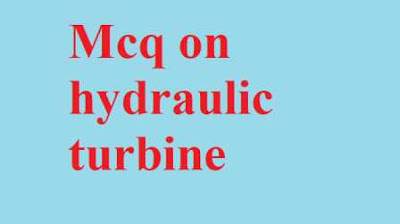### Mcq on Hydraulic turbine (objective questions and answers)

In mechanical engineering mcq website today we solving hydraulic machine subject multiple choice questions Mcq on Hydraulic turbine (objective questions and answers)

Guys this hydraulic turbine in hydraulic machine with answer is important for all competitive exam so without wasting time start Mcq on hydraulic turbine.

# Mcq on Hydraulic turbine (objective questions and answers)Mcq on Hydraulic turbine (objective questions and answers)

Hydraulic turbine mcq - 1

Hydraulic turbine is a .........

A. Positive displacement machine
B. Rotodynamic machine
C. Both A and B
D. None of these

Hydraulic turbine mcq - 2
In hydraulic turbine ............. process take place.

A. Compression
B. Expansion

Hydraulic turbine mcq - 3
Hydraulic turbine is convert the .........

A. Kinetic energy into potential energy
B. Kinetic energy into mechanical energy
C. Potential energy into mechanical energy
D. Mechanical energy into kinetic energy

Hydraulic turbine mcq - 4
Which of the following are types Hydraulic turbine ?

A. Reaction turbine
B. Impulse turbine
C. Both A and B
D. None of these

Hydraulic turbine mcq - 5
Hydraulic turbine is a power ......... hydraulic machine.

A. Genrating
B. Absorbing
C. Cutting
D. None of these

Hydraulic turbine mcq - 6
Hydraulic turbine is reversed to

A. Condenser
B. Hydraulic Pump
C. Compressor
D. Blower

Hydraulic turbine mcq - 7
In hydraulic turbine rotating element is .......

A. Impeller
B. Rotor
C. Disc
D. Roller

Hydraulic turbine mcq - 8
Hydraulic turbine efficiency is ......... than hydraulic pump.

A. More
B. Same
C. Less
D. None of these

Hydraulic turbine mcq - 9
Hydraulic turbine is .......... efficient than hydraulic pump.

A. More
B. Same
C. Less
D. Can't say

Hydraulic turbine mcq - 10
Which principle is used in hydraulic turbines ?

A. Newton zero law
B. Newton first law
C. Newton second law
D. Newton fourth law

Hydraulic turbine mcq - 11
In hydraulic turbine inlet energy is greater than the outlet energy.

A. True
B. False

Hydraulic turbine mcq - 12
Hydraulic turbine convert kinetic energy into mechanical energy.

A. True
B. False

Hydraulic turbine mcq - 13
Hydraulic pump required ......... maintenance than hydraulic turbine.

A. More
B. Same
C. Less
D. None of these

Hydraulic turbine mcq - 14
Hydraulic machines fall under wahich category ?

A. Condenser
B. Pulverizers
C. Rotodynamic machinery
D. Kinetic energy

Hydraulic turbine mcq - 15
In hydraulic turbine pressure is ......... during operation time.

A. Increased
B. Reduced
C. Not change
D. Can't say

Hydraulic turbine mcq - 16
Which types of turbine is Francis turbine ?

A. Impulse turbine
B. Reaction turbine
C. Both A and B
D. None of these

Hydraulic turbine mcq - 17
Which types of turbine is Pelton wheel  turbine ?

A. Impulse turbine
B. Reaction turbine
C. Both A and B
D. None of these

Hydraulic turbine mcq - 18
Which law is used in hydraulic turbine ?

A. Newton zero law
B. Newton first law
C. Newton second law
D. Newton fourth law

Hydraulic turbine mcq - 19
Which types of turbine is propeller turbine ?

A. Impulse turbine
B. Reaction turbine
C. Both A and B
D. None of these

Hydraulic turbine mcq - 20
Which types of turbine is Kaplan's turbine ?

A. Impulse turbine
B. Reaction turbine
C. Both A and B
D. None of these

Hydraulic turbine mcq - 21
kaplan turbine is a ..........

D. None of these

Hydraulic turbine mcq - 22
Pelton wheel turbine is a ..........

D. None of these

Hydraulic turbine mcq - 23
Propeller turbine is a ..........

D. None of these

Hydraulic turbine mcq - 24
Francis's turbine is a ..........

D. None of these

Hydraulic turbine mcq - 25
kaplan turbine is a ..........

A. Low discharge turbine
B. Medium discharge turbine
C. High discharge turbine
D. None of these

Hydraulic turbine mcq - 26
Pelton wheel turbine is a ..........

A. Low discharge turbine
B. Medium discharge turbine
C. High discharge turbine
D. None of these

Hydraulic turbine mcq - 27
Propeller turbine is a ..........

A. Low discharge turbine
B. Medium discharge turbine
C. High discharge turbine
D. None of these

Hydraulic turbine mcq - 28
Francis turbine is a ..........

A. Low discharge turbine
B. Medium discharge turbine
C. High discharge turbine
D. None of these